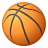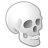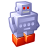# Getting object attributes as values for the rule file

2945
7
Jump to solution
03-22-2013 05:21 AMNew Contributor
Hi there, just one question from a newbie;

I want to use my building height from my object attribute (from shapefile) to recalculate/estimate the number of floors in the building.
To do this I use:

attr BUILDING_H = 0.0 (this works, I now can use BUILDING_H to extrude etc.)

but as soon as I try something like:

numFloors = floor(BUILDING_H/3) it doesn't work anymore since in the rule file BUILDING_H is 0.0 obviously.

Now I tried:

attr Height = getObjectAttr(BUILDING_H) but this doesn't work.

How do I get the attribute to be useable for calculations in the rule file?

cheers
Niek
Tags (3)
1 Solution

Accepted SolutionsNew Contributor III
`//Create some attributes to store data attr BUILDING_H = 0.0 attr HEIGHT = 0.0  MyRule-->     //within a rule: set the Height attr to the value of BUILDING_H,      //assuming BUILDING_H is mapped to a map layer     set(HEIGHT, BUILDING_H)       //use a value of an attr, in this case HEIGHT that was just set above      extrude(world.y, HEIGHT)  //attribute to store number of floors attr numberOfFloors=0  //numFloors is a Function, with the value BH passed to it //case statements work as if/elif/else statements,  //else can be used to catch in this case a building height of 0, //since we only calculate if a building height is over 0,  //if the building height is over 120(units) we divide by 4 instead of three.. if its over 0 but less than 120 we divide by 3  numFloors(BH) =          case BH>120:             floor(BH/4)         case BH > 0:             floor(BH/3)         else:             0  MyRule -->     //here we pass BUILDING_H to our Function numFloors     //the value returned from the calculation is passed to the attribute numberOfFloors,      //functions have funky syntax.. i know..     //attr numberOfFloors value can now be used elsewhere in the rule      set (numberOfFloors, numFloors(BUILDING_H))    `

Does this help?
7 RepliesNew Contributor III
`//Create some attributes to store data attr BUILDING_H = 0.0 attr HEIGHT = 0.0  MyRule-->     //within a rule: set the Height attr to the value of BUILDING_H,      //assuming BUILDING_H is mapped to a map layer     set(HEIGHT, BUILDING_H)       //use a value of an attr, in this case HEIGHT that was just set above      extrude(world.y, HEIGHT)  //attribute to store number of floors attr numberOfFloors=0  //numFloors is a Function, with the value BH passed to it //case statements work as if/elif/else statements,  //else can be used to catch in this case a building height of 0, //since we only calculate if a building height is over 0,  //if the building height is over 120(units) we divide by 4 instead of three.. if its over 0 but less than 120 we divide by 3  numFloors(BH) =          case BH>120:             floor(BH/4)         case BH > 0:             floor(BH/3)         else:             0  MyRule -->     //here we pass BUILDING_H to our Function numFloors     //the value returned from the calculation is passed to the attribute numberOfFloors,      //functions have funky syntax.. i know..     //attr numberOfFloors value can now be used elsewhere in the rule      set (numberOfFloors, numFloors(BUILDING_H))    `

Does this help?New Contributor
Yes it does, thanks a lot!

I see that it has a lot to do with me getting to understand the cga language 😉

Cheers,
NiekNew Contributor
`//Create some attributes to store dataattr BUILDING_H = 0.0attr HEIGHT = 0.0MyRule-->    //within a rule: set the Height attr to the value of BUILDING_H,     //assuming BUILDING_H is mapped to a map layer    set(HEIGHT, BUILDING_H)     //use a value of an attr, in this case HEIGHT that was just set above     extrude(world.y, HEIGHT)//attribute to store number of floorsattr numberOfFloors=0//numFloors is a Function, with the value BH passed to it//case statements work as if/elif/else statements, //else can be used to catch in this case a building height of 0,//since we only calculate if a building height is over 0, //if the building height is over 120(units) we divide by 4 instead of three.. if its over 0 but less than 120 we divide by 3numFloors(BH) =         case BH>120:            floor(BH/4)        case BH > 0:            floor(BH/3)        else:            0MyRule -->    //here we pass BUILDING_H to our Function numFloors    //the value returned from the calculation is passed to the attribute numberOfFloors,     //functions have funky syntax.. i know..    //attr numberOfFloors value can now be used elsewhere in the rule     set (numberOfFloors, numFloors(BUILDING_H))`

Does this help?

Well, I thought it would work now since what you suggested  makes a lot of sense, but... Now BUILDING_H is still not passed on to the attr HEIGHT... Even if I "set(HEIGHT,BUILDING_H)" and then "print(HEIGHT)" it doesn't result in anything. it looks as if the source of BUILDING_H, which is an object attribute, still doesn't allow BUILDING_H to be used in the script (except for the extrude functions etc.)

`// Thisattr BUILDING_H = 0.0attr HEIGHT = 0.0@StartruleHeightSet--> set(HEIGHT, BUILDING_H)  print(HEIGHT)Lot-->extrude(world.y,BUILDING_H)Massattr NumberOfFloors = 0numFloors(BH) =         case BH>120:            floor(BH/4)        case BH > 0:            floor(BH/3.5)        else:            0countFloors-->set (NumberOfFloors, numFloors(HEIGHT))  print(NumberOfFloors) // doesn't result in anyting... `

sorry if i'm a bit of a retard on this 😛Frequent Contributor II
Hi,

did you set the attr source to the object attr ?

in the Inspector, check if behind the attr it says (rule) or (object).

if it says rule, then change the attr source with the little icon right next to it, a bit left. the icon looks like a little black tringle pointing into a white rectangle.New Contributor III
As Matthias says..

Attributes of generated Shapes can recieve their input from a number of sources.
These sources can be changed via the little black arrows next to the attribute values.

Clicking the black arrow with a white square gives access to the Attribute Connection Editor.
If you modify a value of an attribute manually the value in the Attribute Connection Editor is automatically customized to User-defined value so any thing a script/rule does is ignored.
If you expected the script/rule to provide the values to the attribute then make sure its set to Rule-defined value.

My assumption in the example was that your "BUILDING_H" attribute was being driven by some other kind of input, so I was demonstrating how to pass values around to other attributes and to functions as well as how to access them for example the extrude() cga.

I have a question..

What drives your "BUILDING_H" attribute value (what provides its value)?

For example:
Is it connected to a map layer?
Have you set it manually, making it a User-defined value?New Contributor
Hi and thanks again for the support,

my attr BUILDING_H is an attribute that is in the attribute table of a shape file that I imported.

This shapefile contains the lots on which the buildings are generated  and information such as building height and roof slopes etc.

I would like to use this data to do some calculations that have an effect on the final shape of the building but so far I am unable to
use attributes from an object in my script except for the extrude function.

cheers,

NiekFrequent Contributor II